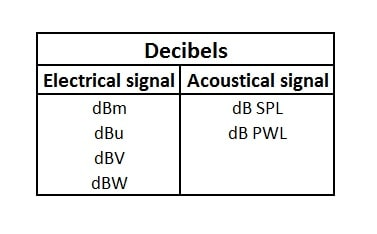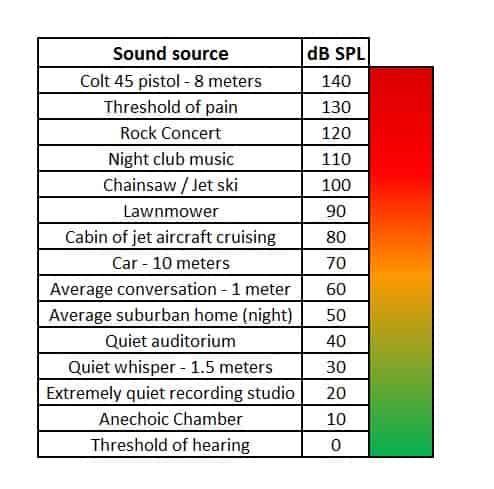## How to calculate decibels and how to interpret their values

The dBu decibel, and the other types also, are often a misunderstood unit of measurement, so let’s learn how to calculate decibels. When people hear about decibels, they immediately think about loudness, which is somewhat incorrect. Well, what is a decibel, you might ask ? The decibel describes a ratio between two quantities, which most often, relate to power. The core unit is a Bel, decibel is actually 0.1 Bels, aka dB. The dB works on a logarithmic scale. This is a good thing, because our ears have a logarithmic sensitivity and for lower db values, there is no need for lots of digits.

### Decibels as a power ratio

We can define a Bel as a logarithm of an electrical power, acoustical power, or any other power ratio.

For example, the relationship between 2 power values P1 and P2, in Bels :

• Bel = log (P1 / P0) .
• dB = 1/10 Bel .
• dB = 10 * log (P1 / P0) .

Let’s give a practical example on how to calculate decibels using watts :

• What is the ratio in dB of 10 watts to 5 watts ?
• dB = 10 * log (10 / 5) = 10 * log(2) = 10 * 0.3 = 3 .
• What is the ratio in dB of 100 watts to 10 watts ?
• dB = 10 * log (100 / 10) = 10 * log(10) = 10 * 1 = 10 .

These ratios I chose intentionally, because they reveal values which are significant and makes interpreting easier :

• Whenever one power is double the other, it is 3 dB greater. 4 times would be 6 dB, 8 times would be 9 dB an so on. If the power is a half the other, we are talking about 3 dB less (-3 dB).
• Whenever one power is 10 times the other, it is 10 dB greater. If it is 1/10 the power, it is 10 dB less (-10 dB).

### Decibels as voltage ratio

We have used the decibel to express power ratios (watts), but it can also be used to express voltage ratios (volts). The decibel relationship of power ratios is not the same for voltage ratios. If we got two voltage values, E1 and E2, we can calculate the relationship between the 2 values (in dB), using the following formula :

• dBvolts = 20 * log (E1 / E0) .

Let’s use the same examples as above on how to calculate decibels, but instead of watts we use voltages :

• What is the ratio in dB of 10 volts to 5 volts ?
• dB = 20 * log (10 / 5) = 20 * log(2) = 20 * 0.3 = 6 .
• What is the ratio in dB of 100 volts to 10 volts ?
• dB = 20 * log (100 / 10) = 20 * log(10) = 20 * 1 = 20 .

For voltages we can draw the following conclusions :

• Whenever one voltage is double the other, it is 6 dB greater. 4 times would be 12 dB, 8 times would be 18 dB an so on. If the voltage is a half the other, we are talking about 6 dB less (-6 dB).
• Whenever one voltage is 10 times the other, it is 20 dB greater. If it is 1/10 the voltage, it is 20 dB less (-20 dB).

### Types of decibels

Why is it that when we double the power, we get a 3 db increase, but when we double the voltage we get a 6 db increase ? This is because of the relationship between power and voltage.

P = E2 / R .

P = Power in watts ; E = voltage in volts and R = resistance in ohms.

As you can see power is proportional to voltage squared. When you double the voltage, you get 4 times the power.

We’ve talked about decibels and how they relate to volts and watts, but where is the loudness I’m used to talk about when I mention decibels ? That’s because decibels relate to 2 things : electrical signals and acoustical signals. Regarding these 2 categories, there are several types of decibels which we will enumerate and explain in the following part of this article.### dBm

The term dBm expresses an electrical power level, which has the reference at 1 milliwatt. This means that 0 dBm = 1 milliwatt, and it has no direct correlation to voltage or impedance. This unit of measurement was an industry standard in the 1940’s. When the unit was first devised, it was mainly used to measure 600 ohm telephone line. Because the power is always 0.001 watts, and the impedance was 600 ohms, this meant that the voltage across the system was exactly 0.775 V rms. For this reason, many people confused the reference of 0 dBm to be equal to 0.775 V. This is not the case, and it is only true when the impedance is 600 ohm.

Example : The maximum output of a preamplifier is + 30 dBm.

• +10 dB increases the output tenfold. So :
• 0 dBm = 0.001 watts.
• +10 dBm = 0.01 watts.
• +20 dBm = 0.1 watts.
• +30 dbm = 1 watt.
• Another way is to use the formula : dB = 10 * log (P1 / P0) .
• 30 = 10 * log (P1 / 0.001)  =>  P1 = 1 watt.

### dBu decibel

The dB scale is always about a ratio, so how to calculate decibels of different types? It all depends on the different points of reference (0 dB mark). dBm was all about power and had the reference point measured in watts. The problem is that many of the equipment used in the audio world, are not that concerned with the power levels but are very sensitive to voltage levels. For this reason, a new unit of measuring was devised, the dBu decibel, which has the reference point of 0 dBu = 0.775 volts. The value was chosen to avoid confusion with the dBm standard. So for a 600 ohm load, both dBm and the dBu decibel, will have the same values.

Example : The maximum output of this preamplifier is +20 dBu.

• +20 dB increases the output tenfold (because we are talking about volts now). So :
• 0 dBu = 0.775 V
• +20 dBu = 7.75 V
• Another way is to use the formula : db = 20 * log (E1 / E0) .
• 20 = 20 * log (E1 / 0.775)  => E1 = 7.75 V

### dBv and dBV

As you might of guess dBV represents a ratio of voltages. The reference voltage is 1 volt RMS. You’re probably wondering if the lower case variant (dBv) is the same as the upper case (dBV). The answer is no. Because of some different convenience factors in the past, dBv describes voltage values just like the dBu decibel. This means that dBv has a reference value of 0.775 V. Just to recap :

• dBv is the same as dBu, with 0 dBv = 0.775 volts.
• dBV has a voltage reference of 0 dBV = 1 volt.

Example : The nominal output level is +4 dBv / dBV. We will use the same formula as for dBu.

• For dBv : 4 = 20 log (E1 / 0.775)  => E1 = 1.23 volts.
• For dBV : 4 = 20 log (E1 / 1)  => E1 = 1.6 volts.

### dBW

As for this type of decibel you probably guess correctly again, it has a power related reference. Just like dBm, but the only difference is the wattage reference. Since dBm is very useful in describing low power outputs, for example microphones or signal processors, it is not so suitable for high power ratings (for power amplifiers). In that case, if dBm has a reference of 0 dBm = 0.001 watts, dBW has a reference of 0 dBW = 1 watt.

### How to design loudspeakers - video courses

This means that :

• 100 W amplifier <=> 20 dBW amplifier  // 10 * log (100 / 1) = 20 dBW
• 500 W amplifier <=> 27 dBW amplifier  // 10 * log (500 / 1) = 27 dBW
• 1000 W amplifier <=> 30 dBW amplifier  // 10 * log (1000 / 1) = 30 dBW

### dB SPL

This is the acoustical part of how to calculate decibels. It describes the sound pressure, since SPL means sound pressure level. This is somewhat related to the voltage ratios. Another term for voltage is electromagnetic force, or EMF. The force of air pressing against the resistance of the eardrum or microphone diaphragm, is analogous to the force of a battery pushing electrons against the resistance of a circuit. Therefore, just like dBvolts , the sound pressure level ratio equation is the following :

• dBSPL = 20 * log (P1 / P0) . Where P1 and P0 are sound pressures (unit of measurement N/m2).

Now that we learned how to calculate decibels SPL we can draw some conclusions. This equation tells us that for every +6 dB, the sound pressure is doubled and for +20 db the sound pressure is ten times greater. But how do we perceive SPL? Well, it’s not exactly like on paper. A mere +3 dB difference is barely perceived as louder and to perceive a sound twice as loud, it should be +10 dB higher. Loudness is a subjective quantity and depends on frequency and absolute sound level.

Just like any other decibel types, dB SPL has a reference. This is 0 dB SPL, also known as the threshold of hearing. For a young undamaged ear, this should be perceived as complete silence and is measured at 0.000002 N/m2. Here is a scale of common sources of sound, with their approximate dB SPL rating:### dB PWL

This type of decibel measures acoustical power, which is expressed in acoustical watts. Since this refers to a power ratio, it uses the same formula like dBm. So here is how to calculate decibels PWL :

• dBPWL = 10 * log (P1 / P0).

Acoustic power is used when calculating the reverb time of an enclosed space, or the efficiency of a loudspeaker. However, speaker manufacturers will more likely quote a dB SPL efficiency rating, because it relates more directly to perceived loudness. Regarding the relationship between electrical watts (dBW) and acoustical watts (dB PWL) there is no obvious rule. However, if you take a closer look to the actual values, you can see how inefficient a speaker system actually is. For example, if a loudspeaker is fed 20 dBW, it might generate as little as 10 dB PWL. Translating this into watts, means that feeding 100 watts into a loudspeaker, might generate as little as 10 W of acoustical power. This would indicate an efficiency of 10%, which is actually pretty high for a loudspeaker in a bass reflex enclosure.

### Conclusion

We now know that regardless of the type of decibel we are using, there is no stand alone value. There will always be a point of reference when we are talking about decibels, and depending on the type, different reference points are taken. Regarding how to calculate decibels, you will first need to know if it is a power ratio or a voltage ratio and apply the appropriate formula.

#### References

1. The Sound Reinforcement Handbook 2nd Edition by Gary Davis and Ralph Jones (Yamaha, 1988). (Amazon affiliate link)